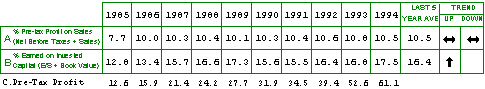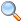Clubs Webinars Investing SSG Tutorial Blog

Section 2: Evaluating Management

The purpose of this section is to help the investor to determine if the company's management is doing a good job, by using two quantitative measurements: the % Pre-Tax Profit on Sales (also known as the "pre-tax profit margin" or PTP) and the % Earned on Invested Capital (also known as the "return on equity" or ROE).

### Entering the DataA. % Pre-Tax Profit on Sales

To calculate the % Pre-Tax Profit on Sales, divide the company's Net Profit Before Taxes by that year's Sales. %PTP is expressed as a percentage, and usually one decimal point is sufficient. Calculate the %PTP for each of the last ten years.

Standard & Poor's reports Pre-Tax Profit in its data sheets. However, users of Value Line may may often be thrown for a loop because this row uses pre-tax profit for the calculation instead of profit after taxes. The rationale is quite simple, though--taxes are entirely determined by outside forces and it would not be fair to blame a company's management if the powers that be in Washington, DC, had again increased taxes. Value Line reports "Net Profit" after taxes, so additional calculations are necessary.

Value Line also reports the company's Tax Rate, so you can figure the Pre-Tax Profit using the following formula:

```
Net Profit
______________  =  Pre-Tax Profit
(1 - Tax Rate)```
(Instead of dividing the Net Profit by the Tax Rate, you're dividing by the Non-Taxed Rate, to give you the amount the company earned as profit before it paid any taxes.) You can probably save a step on the calculator if you can do the subtraction for the denominator of the equation in your head. Think of the percentages as integers--100% minus 30.1% (if the tax rate is 30.1%), results in 69.9%, and use 0.699 when you do the division.

It may be helpful to create a third row in this section, "Row C", to record the actual Pre-Tax Profit for each year that you calculate in this way, as in the above example.

Now you can continue to divide the Pre-Tax Profit by the Sales in the usual way.

B. % Earned on Invested Capital

Calculate the ROE by dividing the Earnings Per Share by the Book Value for each of the past ten years. Again, this figure is expressed as a percentage and it is usually adequate to record just one decimal point in the boxes.

A side note: You may find discrepancies in the book values found in Standard & Poor's and Value Line. This is usually because S&P excludes intangibles in its calculation of book value, reporting Tangible Book Value only.

### Calculating 5-Year Averages

The next step is to calculate the five-year averages for each of these percentages. Add together the last five years of the %PTP and then divide by 5 to determine the average % Pre-Tax Profit on Sales of the last five years. Do the same for the % Earned on Invested Capital.

### Judging the Trends

Finally, you need to determine if any trends exist for both ROE and PTP--has the percentage gone up, down or remained flat? If you have eliminated any years as outliers in Section 1, you may also wish to remove those years from consideration in Section 2 as well. You may also wish to give greater weight to the most recent years, or you can use the 5-year average as a benchmark, comparing the latest year to the average to find a trend. Most investors call the trend "even" if the percentages only vary by just a few tenths of a point from year to year. In the above example, a double-headed arrow indicates an even trend in the % Pre-Tax Profit on Sales.

### Interpreting the Results

The pre-tax profit margin tells you how much profit the company makes on each dollar of sales. The return on equity determines how much profit the company makes compared to the total amount of capital that has been put into the business by its investors.

In optimal conditions, a company would be able to consistently grow its Pre-Tax Profit Margin and Return on Equity. A decreasing profit margin or percentage earned on equity may indicate that a company is having difficulties due to increased competition, rising raw material or labor costs or other problems. If the Return on Equity is decreasing, the company may not be using its capital to best advantage in generating profits for its investors.

Both these figures vary widely by industry, so in looking at these percentages it is helpful to compare the company you're studying with others in its industry. A company with higher margins and return on equity would generally be preferred over a competitor with lower percentages. A high profit margin, on the other hand, invites competition.

### Next